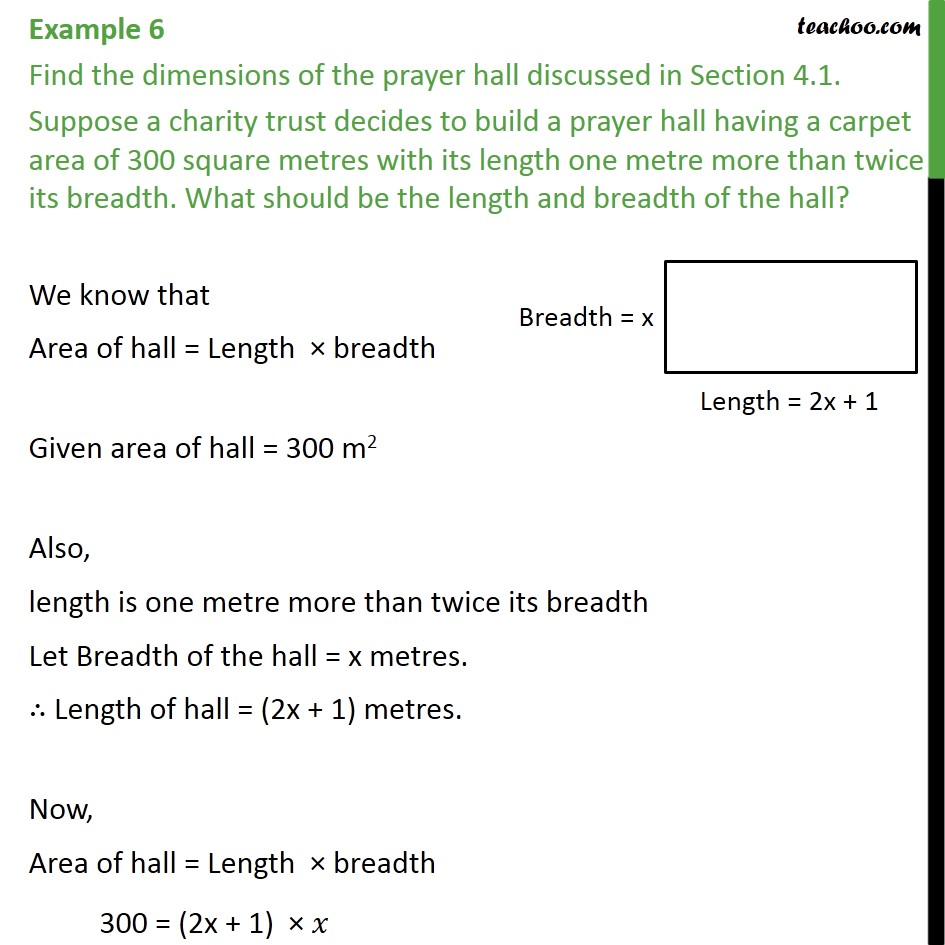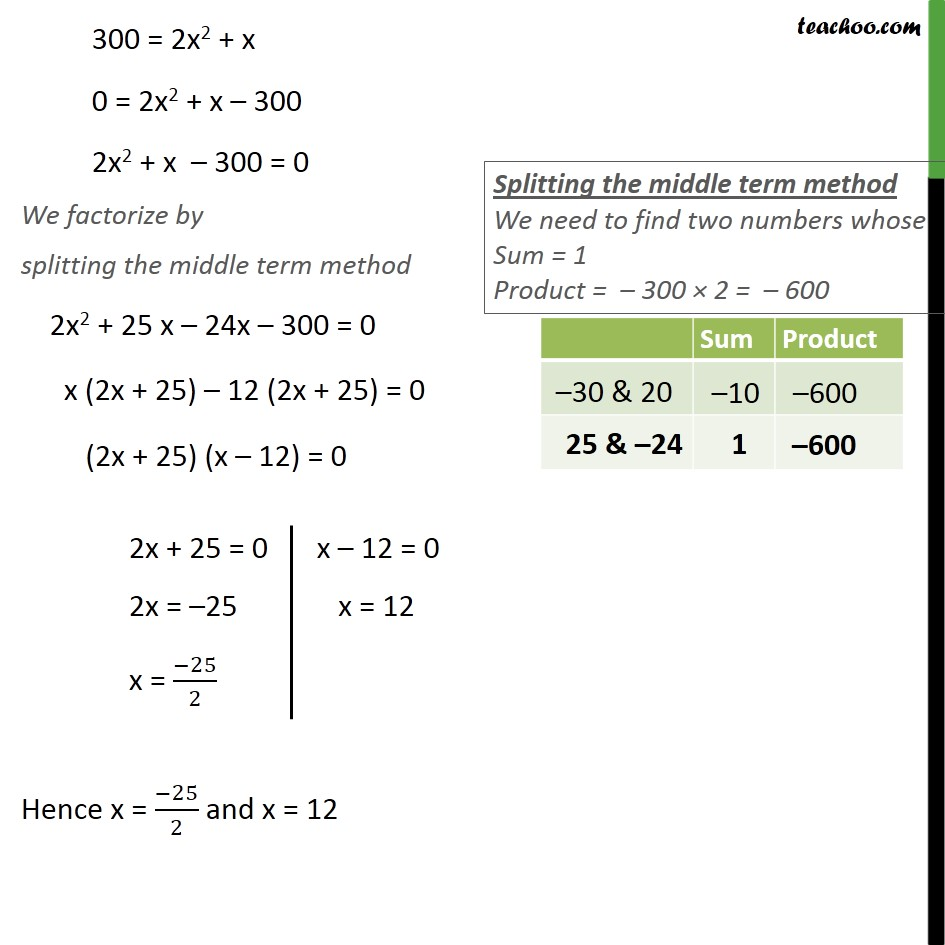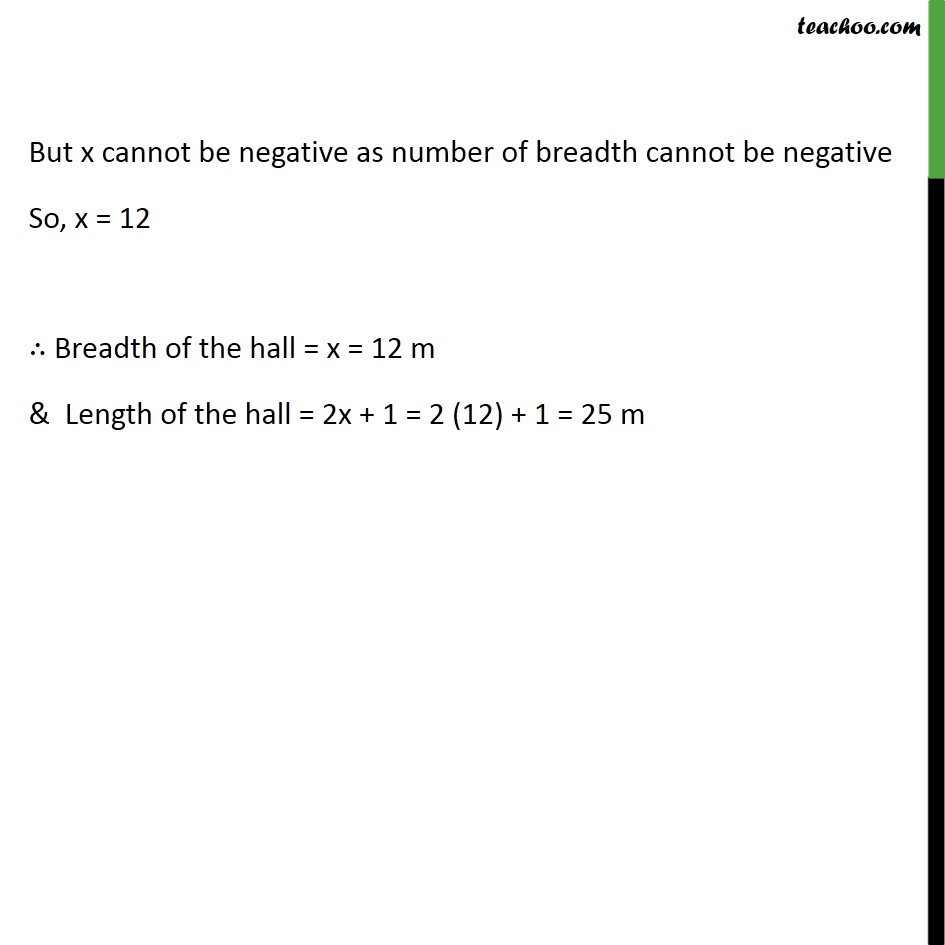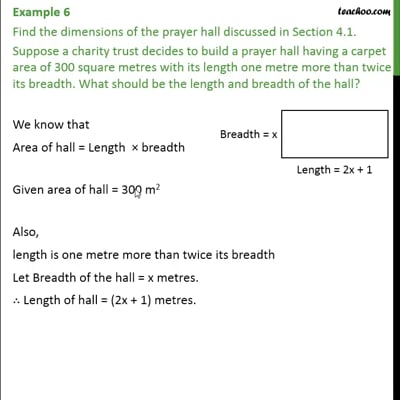Examples

Chapter 4 Class 10 Quadratic Equations (Term 2)
Serial order wiseThis video is only available for Teachoo black users

### Transcript

Example 6 Find the dimensions of the prayer hall discussed in Section 4.1. Suppose a charity trust decides to build a prayer hall having a carpet area of 300 square metres with its length one metre more than twice its breadth. What should be the length and breadth of the hall? We know that Area of hall = Length breadth Given area of hall = 300 m2 Also, length is one metre more than twice its breadth Let Breadth of the hall = x metres. Length of hall = (2x + 1) metres. Now, Area of hall = Length breadth 300 = (2x + 1) 300 = 2x2 + x 0 = 2x2 + x 300 2x2 + x 300 = 0 We factorize by splitting the middle term method 2x2 + 25 x 24x 300 = 0 x (2x + 25) 12 (2x + 25) = 0 (2x + 25) (x 12) = 0 Hence x = ( 25)/2 and x = 12 But x cannot be negative as number of breadth cannot be negative So, x = 12 Breadth of the hall = x = 12 m & Length of the hall = 2x + 1 = 2 (12) + 1 = 25 m